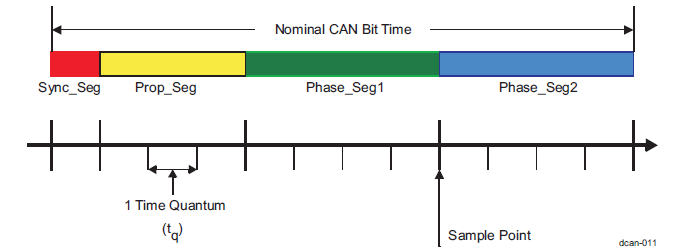• Resolved

# [FAQ] TDA4VM: CAN: How is bit-rate calculated for CAN

Part Number: TDA4VM

1. I see the generated configuration in MCUSW at psdk_rtos_auto_j7_06_02_00_21/mcusw/mcuss_demos/mcal_config/Can_Demo_Cfg/output/generated/soc/j721e/mcu2_1/src/Can_Cfg.c, how do I compute the nominal and data bit rate for MCAN from this?
2. Also, I see the CSL example at psdk_rtos_auto_j7_06_02_00_21/pdk/packages/ti/csl/example/mcan/mcanEvmLoopback/mcan_evm_loopback_app_main.c and see a different structure to set bit rate.

My question is 2 fold, how to compute the bit rate in MCUSW example and how to do it for CSL example. How are these parameters related?

• ### Can bit timing and Sampling pointIn MCUSW - the MCAL CAN driver (psdk_rtos_auto_j7_06_02_00_21/mcusw/mcal_drv/mcal/Can/src/) underneath uses the CAN CSL-FL (psdk_rtos_auto_j7_06_02_00_21/pdk/packages/ti/csl/src/ip/mcan/V1/mcan.c).

Karan Saxena
Also, I see the CSL example at psdk_rtos_auto_j7_06_02_00_21/pdk/packages/ti/csl/example/mcan/mcanEvmLoopback/mcan_evm_loopback_app_main.c and see a different structure to set bit rate.

### Bit rate calculation

bit rate(bits per second) = (CAN clock in Hz) / BRP / (1 + TSEG1 + TSEG2)

where:

CAN clock is functional clock of CAN module (80MHz by default in TDA4)

BRP: Bit rate pre-scalar value

TSEG1, TSEG2: Time segments used to define sampling point for the bit.

TSEG1: Time before the sampling point = Prop_Seg + Phase_Seg1

TSEG2: Time after the sampling point = Phase_Seg2

### Sampling point calculation

Sampling point is the point of time at which the bus level is read and interpreted as the value at that respective time. Typical value of sampling point is between 75-90%.

Sampling Point(%) = (1 + TSEG1) / (1 + TSEG1 + TSEG2)

NOTE: +1 needs to be added in this formula to the BRP, TSEG1 and TSEG2 values while computing the sampling point due to implementation details.

Example: (psdk_rtos_auto_j7_06_02_00_21/pdk/packages/ti/csl/example/mcan/mcanEvmLoopback/mcan_evm_loopback_app_main_k3.c)

``` 784     /* Initialize bit timings
785      * Configuring 1Mbps and 5Mbps as nominal and data bit-rate respectively */
786     bitTimes.nomRatePrescalar   = 0x7U;
787     bitTimes.nomTimeSeg1        = 0x5U;
788     bitTimes.nomTimeSeg2        = 0x2U;
789     bitTimes.nomSynchJumpWidth  = 0x0U;
790     bitTimes.dataRatePrescalar  = 0x1U;
791     bitTimes.dataTimeSeg1       = 0x3U;
792     bitTimes.dataTimeSeg2       = 0x2U;
793     bitTimes.dataSynchJumpWidth = 0x0U;
```

In this configuration calculating the Bit rate: Nominal

TSEG1 = 0x5U +1 = 6

TSEG2 = 0x2U + 1 = 3

BRP = 0x7U + 1 = 8

Nominal Bit rate = 80MHz / 8 / (1 + 6 + 3) = 1Mbps

In this configuration calculating the Bit rate: Data

TSEG1 = 0x3U +1 = 4

TSEG2 = 0x2U + 1 = 3

BRP = 0x1U + 1 = 2

Nominal Bit rate = 80MHz / 2 / (1 + 4 + 3) = 5Mbps

Karan Saxena
I see the generated configuration in MCUSW at psdk_rtos_auto_j7_06_02_00_21/mcusw/mcuss_demos/mcal_config/Can_Demo_Cfg/output/generated/soc/j721e/mcu2_1/src/Can_Cfg.c, how do I compute the nominal and data bit rate for MCAN from this?

As mentioned the MCAL CAN driver too uses the CAN CSL-FL so it follows the same above formulas but there is an implementation difference.

Configuration in MCAL:

``` 92 /* Baud Rate Structure for all configsets */
93 CAN_CONFIG_DATA_SECTION_NON_CONST static Can_BaudConfigType
94     CanConfigSet_CanController_0_CanControllerBaudrateConfig_0 =
95 {
96     1000U,      /* in Kbps */
97     8U,       /* Prop Segement value */
98     6U,          /* Phase Segment 1 */
99     5U,          /* Phase Segment 2 */
100     1U, /* Sync jump width */
101     19U,  /* Sum of all timing parameters  */
102     4U,   /* Controller BRP value for Baud */
103          /* Data phase Baudrate */
104     {
105         5000U,     /* in Kbps */
106         1U,        /* Prop Segement value */
107         1U,           /* Phase Segment 1 */
108         1U,           /* Phase Segment 2 */
109         2U,  /* Sync jump width */
110         2U,  /* Sum of all timing parameters  */
111         4U,   /* Controller BRP value for Baud */
112         180U, /* Specifies the Transceiver Delay Compensation Offset in ns */
113         (boolean)TRUE, /* Specifies if the bit rate switching shall be used */
114     }
115 };
```

In this configuration we see Prop Segment value, Phase Segment 1 & 2. These are the same as we see in the diagram above.

In this configuration calculating the Bit rate: Nominal

TSEG1 = Prop Segment value + Phase Segment 1 = 8 + 6 = 14

TSEG2 = Phase Segment 2 = 5

BRP = 4

Nominal Bit rate = 80MHz / ( (4) * (1 + 14  + 5) ) = 1Mbps

NOTE: Here we do not need to add +1.

In this configuration calculating the Bit rate: Data

TSEG1 = Prop Segment value + Phase Segment 1 = 2

TSEG2 = Phase Segment 2 = 1

BRP = 4

Data Bit rate = 80MHz / ( (4) * (1 + 2 + 1) ) = 5Mbps

Regards,

Karan

• In reply to Karan Saxena:

Great Work Karan !

I can modify the CAN nominal bit rate to 500kb/s from 1Mb/s by using your method, this guide is very helpful for CAN setting, thanks a lot~

Best regard,

Neo